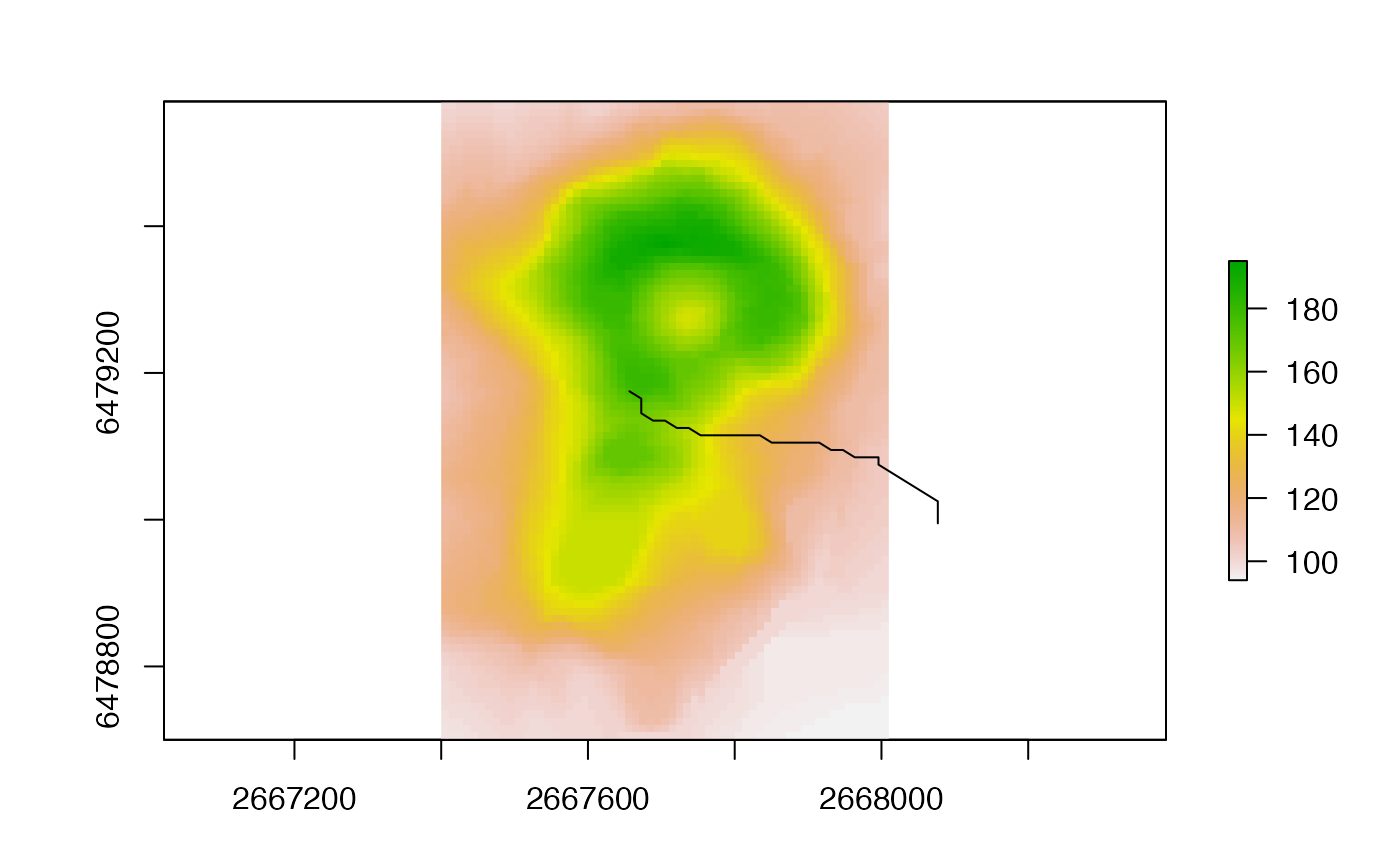Compute the flow path (drainage path) starting at a given point. See package gdistance for more path computations.

flowPath(x, p, ...)

## Arguments

x

RasterLayer of flow direction (as can be created with terrain

p

starting point. Either two numbers: x (longitude) and y (latitude) coordinates; or a single cell number

...

## Value

numeric (cell numbers)

## Author

Ashton Shortridge

## Examples

data(volcano)
v <- raster(volcano, xmn=2667400, xmx=2668010, ymn=6478700, ymx=6479570, crs="+init=epsg:27200")
fd <- terrain(v, opt = "flowdir")
path <- flowPath(fd, 2407)
xy <- xyFromCell(fd, path)
plot(v)
lines(xy)